# Claire and book

After Claire has read the first 5/8 of the book, there are 120 pages left to read. What is the total number of pages of the book?

n =  320

### Step-by-step explanation:Did you find an error or inaccuracy? Feel free to write us. Thank you!Tips to related online calculators
Need help to calculate sum, simplify or multiply fractions? Try our fraction calculator.
Do you have a linear equation or system of equations and looking for its solution? Or do you have a quadratic equation?

## Related math problems and questions:

• Book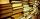Jitka read on holidays book that has 180 pages. In the first week read 45 pages. In the second week she read 15 pages more than the first week. How many pages left to read it yet?
• Book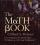John read the book so that it read 30 pages per day. Maxim read 34 pages a day and read it two days earlier than John. How many pages has the book?
• ClarissaClarissa read 1/2 of a book in the morning, and she read some more at night. By the end of the day, she still had 1/3 of the book left to read. How much of the book did Clarissa read at night?
• Janka and Danka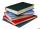Janka and Danka began to read the same day the books. Janka had 276 pages, Danka 204 pages. They agreed that both read every day the same number of pages until theirs books read. A) How many maximum pages they had to read the day to honor their agreement?
• Book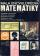To number pages of the thick book was used 4201 digits. How many pages has this book?
• Borrowed book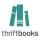Jane must as soon as possible return a borrowed book. She figured that when she read 15 pages a day return book in time. Then she read 18 pages a day and then return the book one day before. How many pages should have a book?
• I think numberI think number.When I add 841 to it and subtract 157, I get a number that is 22 greater than 996. What number I thinking?
• Unknown number 6Determine x if 1/6 of x is equal to 2/5 of the number 24.
• The sum 12The sum of 3 mixed numbers is 20 13/15. two of the numbers are 6 1/3 and 7 5/6. what is the third number?
• Pages of book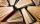Lenka calculated that if every day to read 16 pages of the book, read a book a day earlier than had read only 14 pages a day. How many pages has this book?
• Pages countingThere are pages numbered from 2 to 104 in the book. How many digits have to be printed to number the pages?
• Fractions and mixed numerals(a) Convert the following mixed numbers to improper fractions. i. 3 5/8 ii. 7 7/6 (b) Convert the following improper fraction to mixed number. i. 13/4 ii. 78/5 (c) Simplify these fractions to their lowest terms. i. 36/42 ii. 27/45 2. evaluate following exSusan thought, "If I read 15 pages a day, I will read the whole book in 8 days. “How many pages would she have to read a day if she wanted to finish the book on the 6th day from the start of reading? And how many pages does the book have?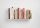Miguel and Kat are reading the same book. Miguel reads three times as many pages of the book as Kat. Together they read 48 pages. Write an equation that can be used to find how many pages Kat read.3/5 trees are apples, cherries are 1/3. 5 trees are pear. How many is the total number of trees?What is the Lcm of 120 15 and 5John Smith is reading a 435-page novel. If he reads the same number of pages per day and the number is a prime number greater than 10, how many pages per day will he read?